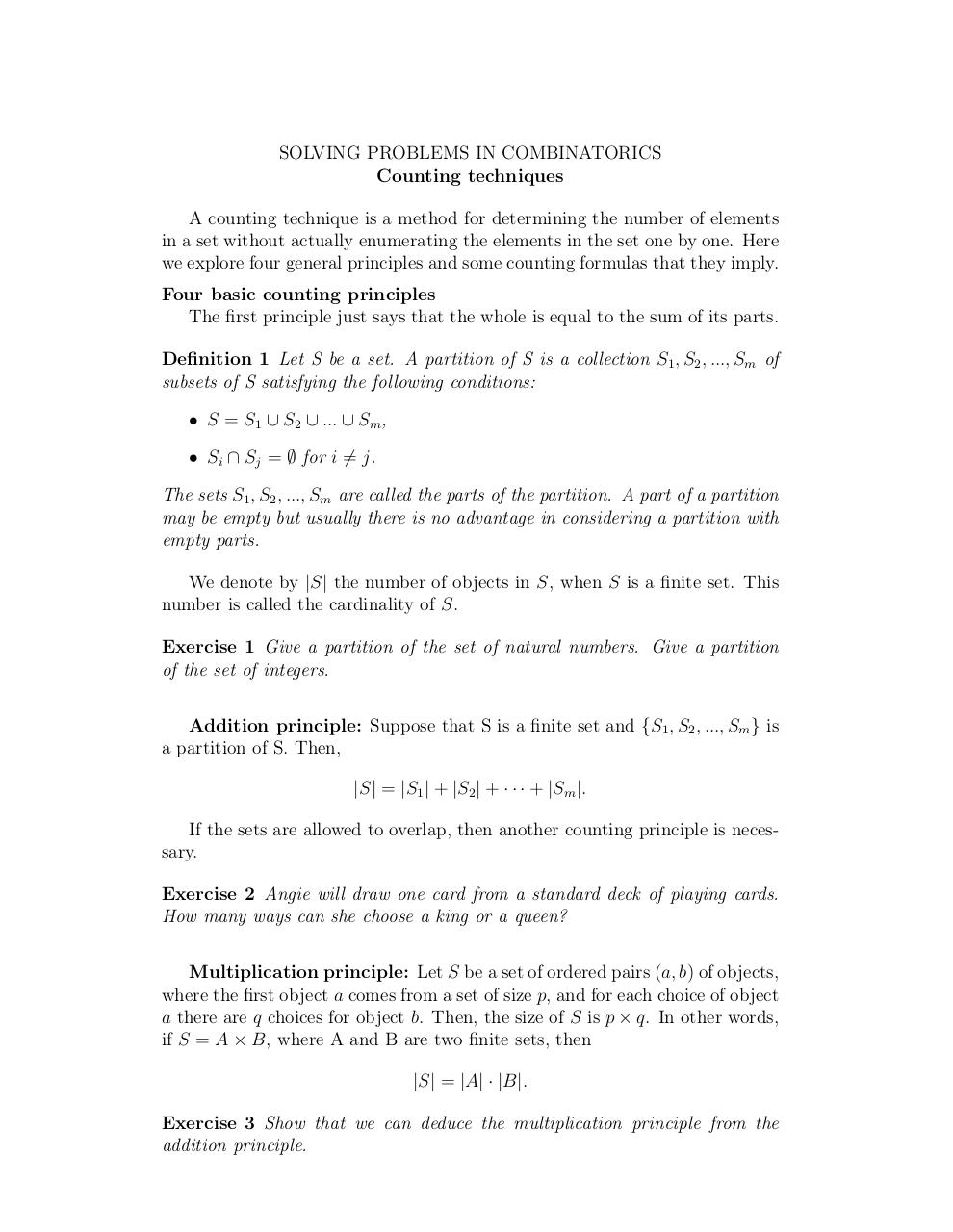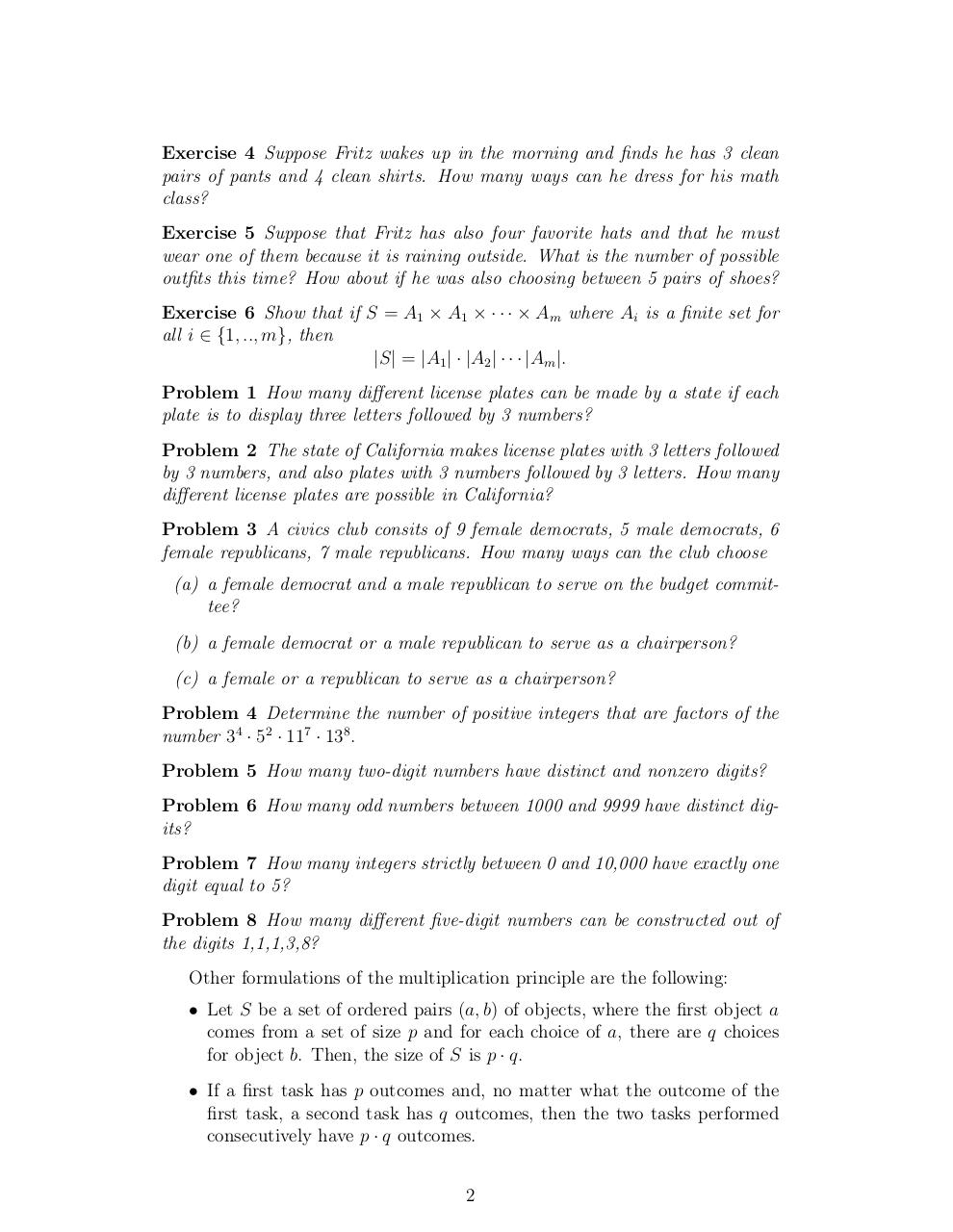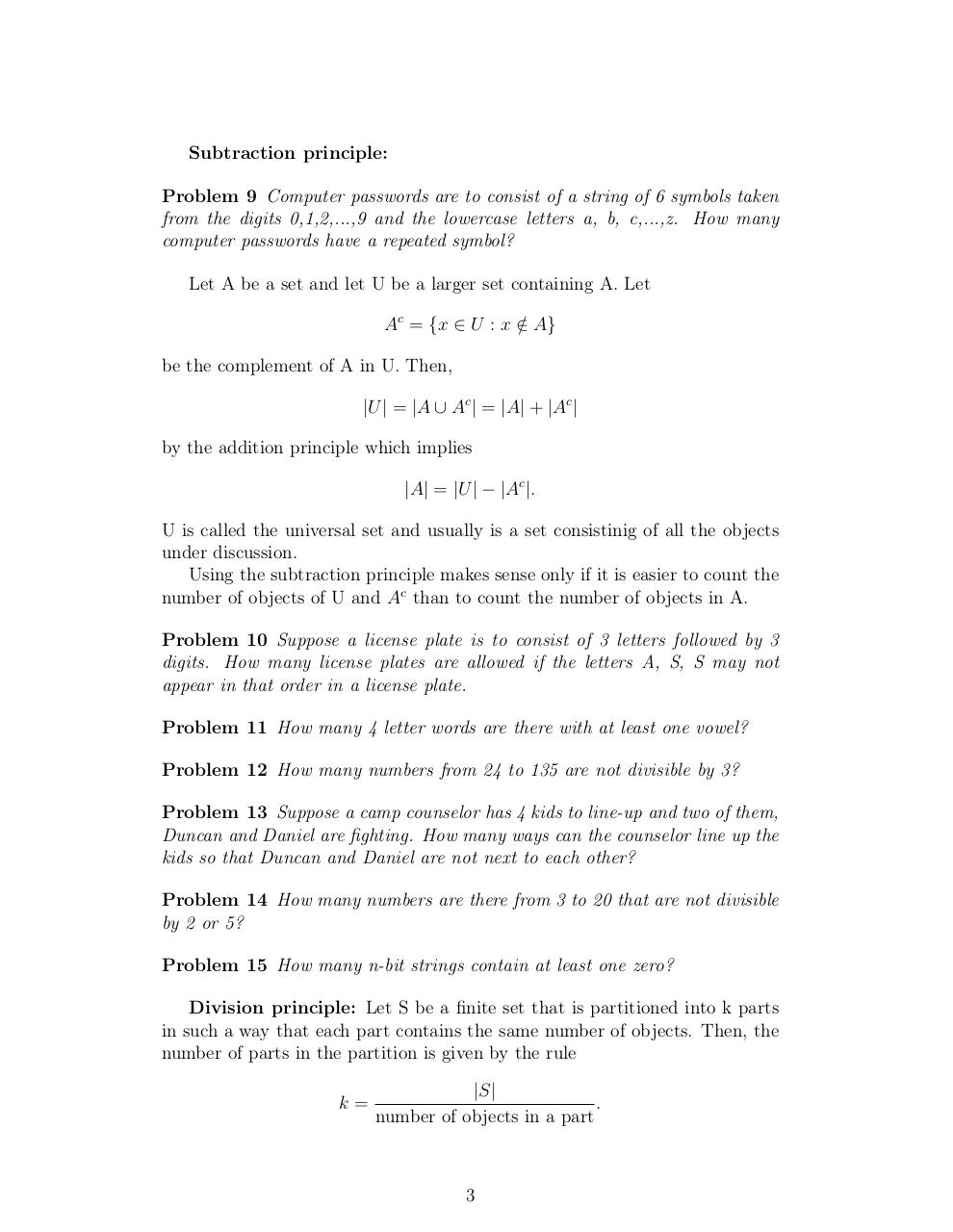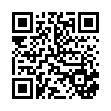# Day 5 Counting Techniques .pdf

### File information

Original filename: Day 5-Counting Techniques.pdf

This PDF 1.4 document has been generated by TeX / pdfTeX-1.40.3, and has been sent on pdf-archive.com on 24/01/2014 at 06:06, from IP address 169.231.x.x. The current document download page has been viewed 784 times.
File size: 103 KB (3 pages).
Privacy: public file

Day 5-Counting Techniques.pdf (PDF, 103 KB)

### Document preview

SOLVING PROBLEMS IN COMBINATORICS
Counting techniques
A counting technique is a method for determining the number of elements
in a set without actually enumerating the elements in the set one by one. Here
we explore four general principles and some counting formulas that they imply.
Four basic counting principles
The first principle just says that the whole is equal to the sum of its parts.
Definition 1 Let S be a set. A partition of S is a collection S1 , S2 , ..., Sm of
subsets of S satisfying the following conditions:
• S = S1 ∪ S2 ∪ ... ∪ Sm ,
• Si ∩ Sj = ∅ for i 6= j.
The sets S1 , S2 , ..., Sm are called the parts of the partition. A part of a partition
may be empty but usually there is no advantage in considering a partition with
empty parts.
We denote by |S| the number of objects in S, when S is a finite set. This
number is called the cardinality of S.
Exercise 1 Give a partition of the set of natural numbers. Give a partition
of the set of integers.
Addition principle: Suppose that S is a finite set and {S1 , S2 , ..., Sm } is
a partition of S. Then,
|S| = |S1 | + |S2 | + · · · + |Sm |.
If the sets are allowed to overlap, then another counting principle is necessary.
Exercise 2 Angie will draw one card from a standard deck of playing cards.
How many ways can she choose a king or a queen?
Multiplication principle: Let S be a set of ordered pairs (a, b) of objects,
where the first object a comes from a set of size p, and for each choice of object
a there are q choices for object b. Then, the size of S is p × q. In other words,
if S = A × B, where A and B are two finite sets, then
|S| = |A| · |B|.
Exercise 3 Show that we can deduce the multiplication principle from the

Exercise 4 Suppose Fritz wakes up in the morning and finds he has 3 clean
pairs of pants and 4 clean shirts. How many ways can he dress for his math
class?
Exercise 5 Suppose that Fritz has also four favorite hats and that he must
wear one of them because it is raining outside. What is the number of possible
outfits this time? How about if he was also choosing between 5 pairs of shoes?
Exercise 6 Show that if S = A1 × A1 × · · · × Am where Ai is a finite set for
all i ∈ {1, .., m}, then
|S| = |A1 | · |A2 | · · · |Am |.
Problem 1 How many different license plates can be made by a state if each
plate is to display three letters followed by 3 numbers?
Problem 2 The state of California makes license plates with 3 letters followed
by 3 numbers, and also plates with 3 numbers followed by 3 letters. How many
different license plates are possible in California?
Problem 3 A civics club consits of 9 female democrats, 5 male democrats, 6
female republicans, 7 male republicans. How many ways can the club choose
(a) a female democrat and a male republican to serve on the budget committee?
(b) a female democrat or a male republican to serve as a chairperson?
(c) a female or a republican to serve as a chairperson?
Problem 4 Determine the number of positive integers that are factors of the
number 34 · 52 · 117 · 138 .
Problem 5 How many two-digit numbers have distinct and nonzero digits?
Problem 6 How many odd numbers between 1000 and 9999 have distinct digits?
Problem 7 How many integers strictly between 0 and 10,000 have exactly one
digit equal to 5?
Problem 8 How many different five-digit numbers can be constructed out of
the digits 1,1,1,3,8?
Other formulations of the multiplication principle are the following:
• Let S be a set of ordered pairs (a, b) of objects, where the first object a
comes from a set of size p and for each choice of a, there are q choices
for object b. Then, the size of S is p · q.
• If a first task has p outcomes and, no matter what the outcome of the
consecutively have p · q outcomes.
2

Subtraction principle:
Problem 9 Computer passwords are to consist of a string of 6 symbols taken
from the digits 0,1,2,...,9 and the lowercase letters a, b, c,...,z. How many
computer passwords have a repeated symbol?
Let A be a set and let U be a larger set containing A. Let
Ac = {x ∈ U : x ∈
/ A}
be the complement of A in U. Then,
|U | = |A ∪ Ac | = |A| + |Ac |
by the addition principle which implies
|A| = |U | − |Ac |.
U is called the universal set and usually is a set consistinig of all the objects
under discussion.
Using the subtraction principle makes sense only if it is easier to count the
number of objects of U and Ac than to count the number of objects in A.
Problem 10 Suppose a license plate is to consist of 3 letters followed by 3
digits. How many license plates are allowed if the letters A, S, S may not
appear in that order in a license plate.
Problem 11 How many 4 letter words are there with at least one vowel?
Problem 12 How many numbers from 24 to 135 are not divisible by 3?
Problem 13 Suppose a camp counselor has 4 kids to line-up and two of them,
Duncan and Daniel are fighting. How many ways can the counselor line up the
kids so that Duncan and Daniel are not next to each other?
Problem 14 How many numbers are there from 3 to 20 that are not divisible
by 2 or 5?
Problem 15 How many n-bit strings contain at least one zero?
Division principle: Let S be a finite set that is partitioned into k parts
in such a way that each part contains the same number of objects. Then, the
number of parts in the partition is given by the rule
k=

|S|
.
number of objects in a part

3#### HTML Code

Copy the following HTML code to share your document on a Website or Blog

#### QR Code### Related keywords